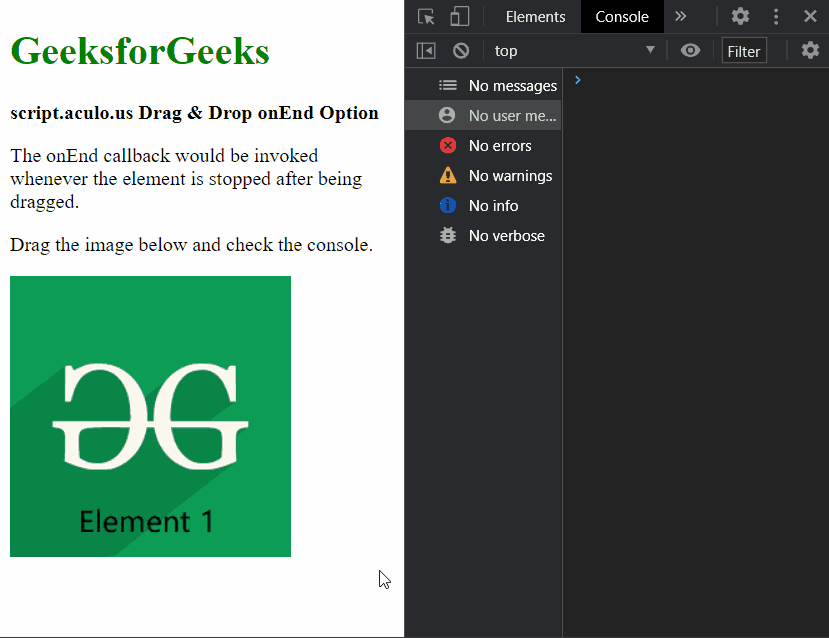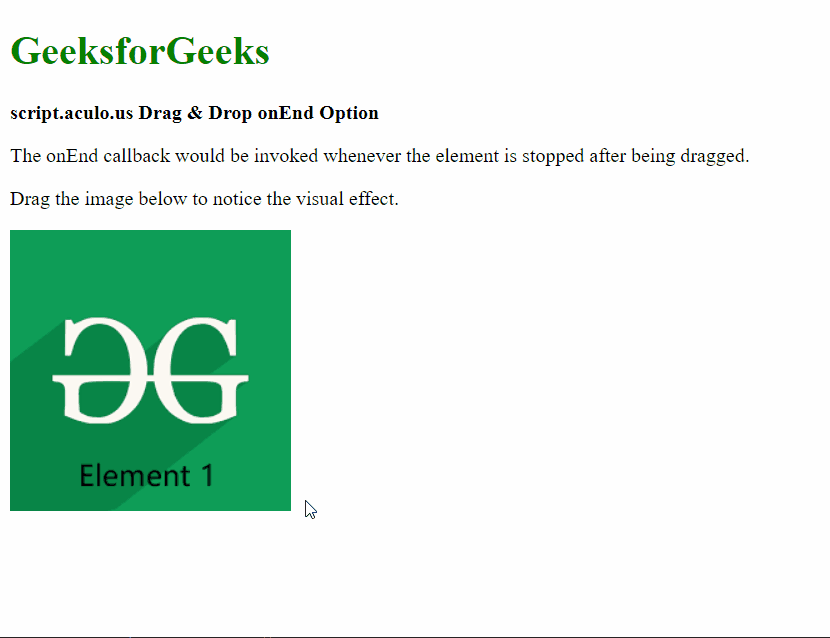# script.aculo.us Drag & Drop onEnd Option

• Last Updated : 25 Dec, 2020

The script.aculo.us library is a cross-browser library that aims at improving the user interface of a website. The Drag & Drop module can be used to make any element drag-gable and also enables them to be dropped in a drop zone.

The onEnd option is used to specify a callback function that would be invoked whenever the dragging of the element ends. The element which is dragged would be passed as the parameter to the function.

Include Scripts:

Step 2: Unzip the files and put the required files (mainly prototype.js and scriptaculous.js) in the current root directory of your folder.

Step 3: Place any image of your choice in the current root directory of your folder, like in the following example elem1.png is used.

Syntax:

`{ onEnd: function }`

Parameters: This option has a single value as mentioned above and described below:

• function: This is a callback function that would be invoked whenever the dragging of an element ends.

The below examples illustrates the use of this option.

Example 1:

## HTML

 ` ` `<``html``> ` `<``head``> ` `  ``<``script` `type``=``"text/javascript"`  `    ``src``=``"prototype.js"``> ` `  `` ` `  ``<``script` `type``=``"text/javascript"`  `    ``src``=``"scriptaculous.js"``> ` `  `` ` ` `  `  ``<``script` `type``=``"text/javascript"``> ` `    ``window.onload = function () { ` ` `  `      ``// Define a function to be used ` `      ``// when the element stops after ` `      ``// being dragged ` `      ``new Draggable('elem1', { ` `        ``onEnd: (elem) => { ` `          ``console.log( ` `            ``"The dragging of the element has stopped" ` `          ``); ` `          ``console.log(elem); ` `        ``} ` `      ``}); ` `    ``}; ` `  `` ` ` ` ` `  `<``body``> ` `  ``<``div``> ` `    ``<``h1` `style``=``"color: green"``> ` `      ``GeeksforGeeks ` `    `` ` `  `` ` `  ``<``strong``> ` `    ``script.aculo.us Drag & ` `    ``Drop onEnd Option ` `  `` ` `   `  `  ``<``p``> ` `    ``The onEnd callback would be ` `    ``invoked whenever the element  ` `    ``is stopped after being dragged. ` `  `` ` `   `  `  ``<``p``> ` `    ``Drag the image below and ` `    ``check the console. ` `  `` ` ` `  `  ``<``img` `id``=``"elem1"` `src``=``"elem1.png"``> ` ` ` ``

Output:Example 2:

## HTML

 ` ` `<``html``> ` ` `  `<``head``> ` `  ``<``script` `type``=``"text/javascript"`  `    ``src``=``"prototype.js"``> ` `  `` ` `  ``<``script` `type``=``"text/javascript"`  `    ``src``=``"scriptaculous.js"``> ` `  `` ` ` `  `  ``<``script` `type``=``"text/javascript"``> ` `    ``window.onload = function () { ` `      ``new Draggable('elem1', { ` ` `  `        ``// Define a function to be used ` `        ``// when the element stops  ` `        ``// to be dragged ` `        ``onEnd: () => { ` `          ``new Effect.Squish('elem1', ` `          ``); ` `        ``} ` `      ``}); ` `    ``}; ` `  `` ` ` ` `<``body``> ` `  ``<``div``> ` `    ``<``h1` `style``=``"color: green"``> ` `      ``GeeksforGeeks ` `    `` ` `  `` ` `  ``<``strong``> ` `    ``script.aculo.us Drag & ` `    ``Drop onEnd Option ` `  `` ` `   `  `  ``<``p``> ` `    ``The onEnd callback would be  ` `    ``invoked whenever the element is ` `    ``stopped after being dragged. ` `  `` ` ` `  `  ``<``p``> ` `    ``Drag the image below to notice ` `    ``the visual effect. ` `  `` ` ` `  `  ``<``img` `id``=``"elem1"` `src``=``"elem1.png"``> ` ` ` ` `  ``

Output:Reference: http://script.aculo.us/

My Personal Notes arrow_drop_up
Recommended Articles
Page :